# How to Programmatically Customize Number Formatting in C# Excel XLSX

Number Format is an important and frequently used feature offered by Microsoft Excel to improve readability, for a unified display of the same information, for data consistency, for easier formula calculations/analysis, and for quick decision-making.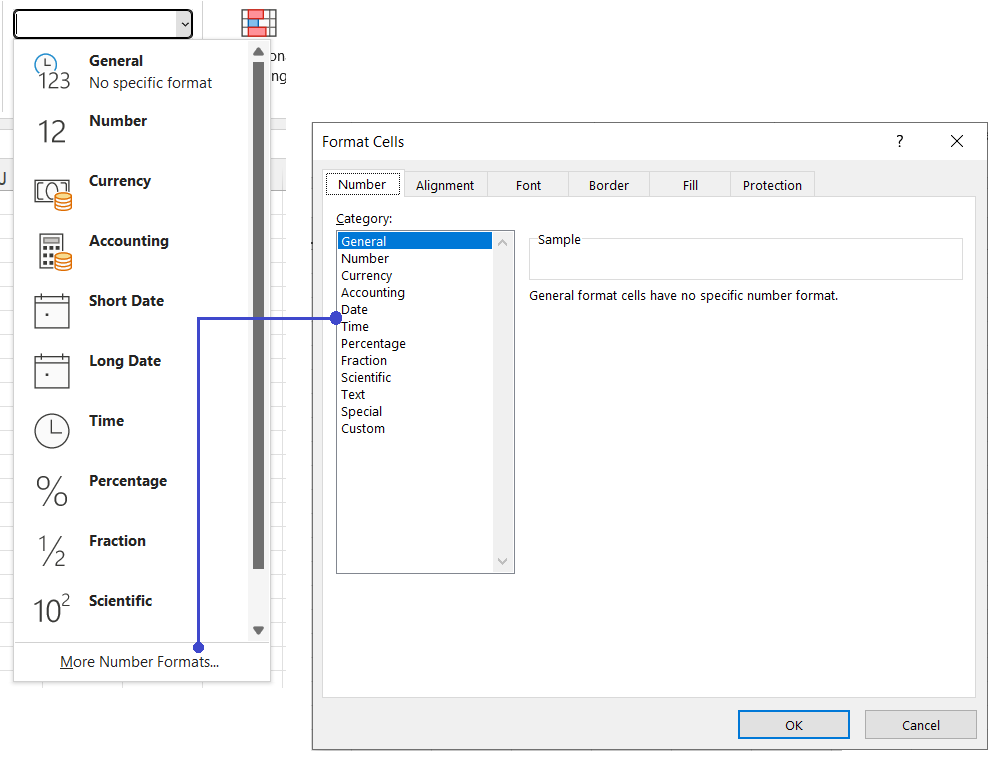Though MS Excel intelligently applies the relevant data format automatically when data is entered manually, populated from a source, or copied from another location such as an HTML table. However, there are situations when the applied format changes the purpose or the nature of the data. For instance:

• numbers stored in some regional formats are stored in General format, thus, are not evaluated correctly in formulas
• identifiers with leading zeros are converted to Number format, therefore, losing the zeros
• dates are converted to their number counterparts, and so on

Such situations require manually setting the correct and relevant Number Format to the data.

In this blog, we will see the popular data scenarios where number formatting is required in an Excel file and how it is applied using GcExcel API for .NET.

## NumberFormat property in GcExcel

GcExcel supports the NumberFormat property with every text object, such as Chart’s Title, DataLabels, Cell/Range, Text Style, Pivot Fields, etc. It accepts a string in the following syntax:

``<format for positive number>;<format for negative number>;<format for zero>;<format for text>``

In most common scenarios, the format code contains one section of the syntax, for instance, "General" or "##.00" and the format is applied to all data types - positive number, negative number, zeros, and text. However, several data scenarios use multiple sections of the syntax separated by a semicolon ( ; ) as covered in the topics listed below:

## Import Dates stored as texts to a Date format

Often numeric or date-type data generated by enterprise applications are stored as texts. This happens mostly with data stored as JSON or CSV. For example:

``````[
. . .
{
"Area": "North America",
"City": "Chicago",
"Category": "Consumer Electronics",
"Name": "Bose 785593-0050",
"Revenue": 92800,
"SalesFrom": "1/10/2022",
"SalesTo": "1/11/2022"
},
. . .
]``````

When such data is loaded into a workbook’s cell, it is stored in General number format. To save it as a date in the generated Excel spreadsheet, apply the number format to the respective data columns after importing data as depicted in the code below:

``````//create a new workbook
var workbook = new GrapeCity.Documents.Excel.Workbook();
workbook.AutoParse = true;

// Get data from json file.
string jsonText = string.Empty;
using (Stream stream = GetResourceStream("json\\DataBinding.json"))
{
}

IWorksheet worksheet = workbook.ActiveSheet;

worksheet.DataSource = new JsonDataSource(jsonText);
worksheet.Columns.NumberFormat = "dd/mm/yyyy";
worksheet.Columns.NumberFormat = "d/m/yy";

// Save to an excel file
workbook.Save("JsonSource.xlsx");``````

The output is shown in the image below: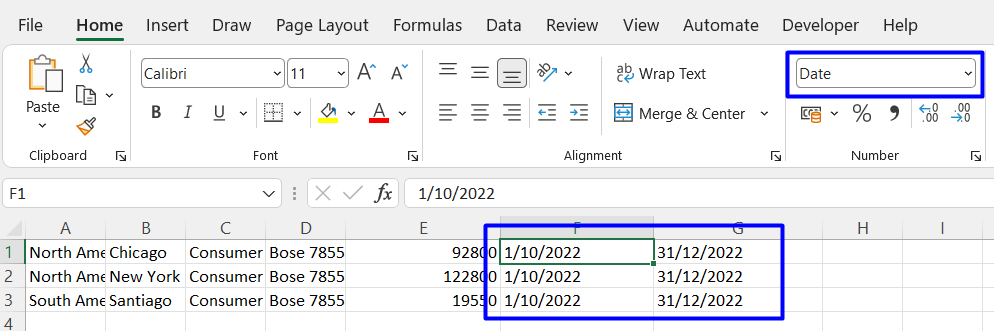## Show big numbers in a format other than scientific format

Excel most likely displays numbers in scientific rather than the number format for numbers longer than 12 digits, such as UPC/GTIN code, ID, etc.

This is because of Excel's 15-digit precision limit, which was implemented to preserve accuracy. To change big numbers from scientific notation to a number, use the number code as depicted in the code below:

``````IWorkbook workbook = new GrapeCity.Documents.Excel.Workbook();
workbook.Worksheets["Big Number"].Range["A1"].Value = "From Data";
workbook.Worksheets["Big Number"].Range["B1"].Value = "Converted to number";

workbook.Worksheets["Big Number"].Range["A2:B2"].Value = 6589423145697;
workbook.Worksheets["Big Number"].Range["B2"].NumberFormat = "0";``````

The following image depicts a big number displayed in Excel with (and without) the number format applied: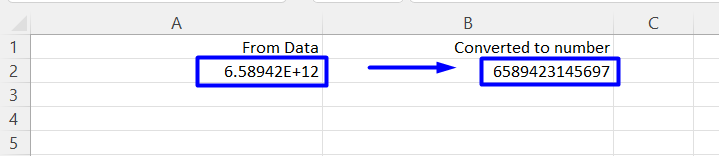Numbers representing entities such as Postal/Zip code, system-generated identifier, etc., starting or ending in zero, such as 00123, 1234.5600, etc., are automatically converted to numbers (by removing the leading and trailing zeros) in Excel. To retain the zeros, number-format them using the placeholder “0” as depicted in the code snippet below:

``````workbook.Worksheets.Add().Name = "Zeros";
workbook.Worksheets["Big Number"].Range["A1"].Value = "From Data";
workbook.Worksheets["Big Number"].Range["B1"].Value = "Converted to number";

workbook.Worksheets["Big Number"].Range["A2"].Value = 00123;
workbook.Worksheets["Big Number"].Range["A2"].NumberFormat = "00000";

workbook.Worksheets["Big Number"].Range["B2"].Value = 1234.5600;
workbook.Worksheets["Big Number"].Range["B2"].NumberFormat = "#.0000";``````

The numbers appear with and without a number format, as shown in the image below: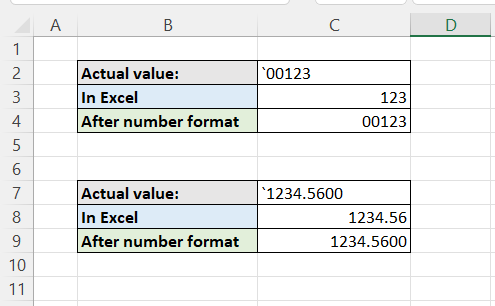## Color Numbers/Text Conditionally

Suppose the dataset contains a mix of positive numbers, negative numbers, and text content - for instance, a survey result with positive or negative ratings and comments. Apart from conditional formatting, Number format can highlight them for quick readability. The number format supports the eight primary colors, Black, Green, Red, White, Blue, Magenta, Yellow, and Cyan. Just add these colors within the square brackets[ ] for the respective number, as shown in the code section below:

``````object[,] data = new object[,]{
{"Name", "City", "Result"},
{"Richard", "New York", 10 },
{"Nia", "New York", 5},
{"Jared", "New York",-6 },
{"Natalie", "Washington",-2 },
{"Damon", "Washington", "Unimpressive" },
{"Angela", "Washington", "Unimpressive" }
};

workbook.Worksheets["Conditional Format"].Range["A1:C7"].Value = data;
workbook.Worksheets["Conditional Format"].Range["C2:C7"].NumberFormat = "General;[Red]-General;;[Blue]General";
workbook.Worksheets["Conditional Format"].Columns.AutoFit();``````

See the “Result” column in the image below: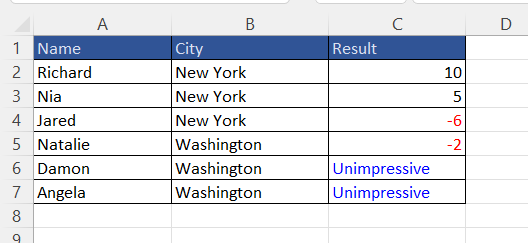## Disguise Numbers with Texts

Data like test scores, Boolean flags, age, etc., are often discrete. For analysis purposes, it is required to replace those numbers with meaningful, categorized, or readable text such as Pass/Fail, True/False, and so on. Besides conditional formatting, formulas & functions, a Number format can be used for this requirement by adding the desired condition within square brackets [ ], as shown below:

``````object[,] data1 = new object[,]{
{"Name", "Subject", "Result"},
{"Richard", "English", 70 },
{"Richard", "Elective-I", 65},
{"Richard", "Science",45 },
{"Richard", "Mathematics",56 },
{"Richard", "Elective-II", 25 },
{"Richard", "Language", 38 },
{"Natalie", "English", 30 },
{"Natalie", "Elective-I", 45},
{"Natalie", "Science",53 },
{"Natalie", "Mathematics",28 },
{"Natalie", "Elective-II", 32 },
{"Natalie", "Language", 69 }
};

workbook.Worksheets["DisguiseNumber"].Range["A1:C13"].Value = data1;
//disguise number without color code
workbook.Worksheets["DisguiseNumber"].Range["C2:C13"].NumberFormat = "[<35]\"Fail\";\"Pass\"";
//disguise number with color code
//workbook.Worksheets["DisguiseNumber"].Range["C2:C13"].NumberFormat = "[<35][Red]\"Fail\";[Green]\"Pass\"";

workbook.Worksheets["DisguiseNumber"].Columns.AutoFit();``````

The image below shows the original data in column C and when disguised as texts in column D.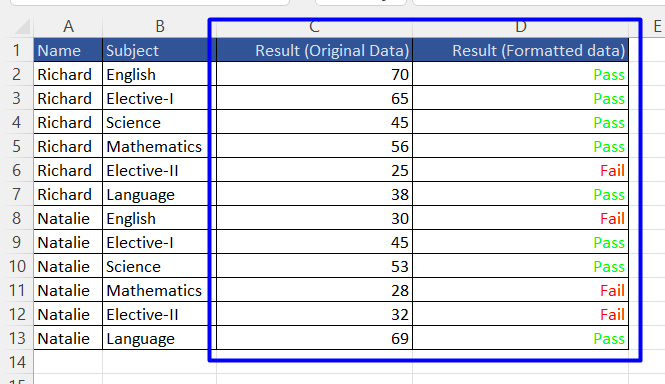## Format Numbers as Thousands, Millions, or Billions

When dealing with large numbers, especially amount/price, or figures such as population, it is common to display them on a shorter scale, such as thousands, million, etc. Large numbers can be formatted to different number scales using Number Format as depicted in the code below:

``````workbook.Worksheets.Add().Name = "NumberScale";

workbook.Worksheets["NumberScale"].Range["A1"].Value = "Number scale Format";
workbook.Worksheets["NumberScale"].Range["A1:D1"].MergeCells = true;

workbook.Worksheets["NumberScale"].Range["A3"].Value = "Number";
workbook.Worksheets["NumberScale"].Range["B3"].Value = "Thousands";
workbook.Worksheets["NumberScale"].Range["C3"].Value = "Million";
workbook.Worksheets["NumberScale"].Range["D3"].Value = "Billion";

workbook.Worksheets["NumberScale"].Range["A4:D4"].Value = 456321456;
workbook.Worksheets["NumberScale"].Range["B4"].NumberFormat = "0.00, \"K\"";
workbook.Worksheets["NumberScale"].Range["C4"].NumberFormat = "\$0.00,, \"M\"";
workbook.Worksheets["NumberScale"].Range["D4"].NumberFormat = "\$#.##,,, \"B\"";``````

The image below shows representing numbers on different scales: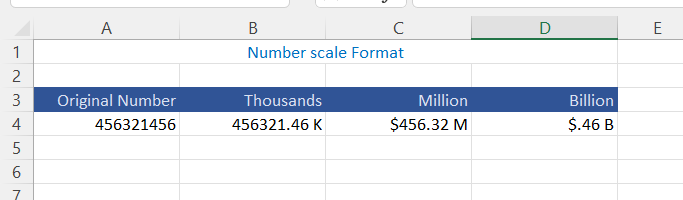## Hide a Cell Value

This is the most naive scenario for a number format. However, it is super cool for situations to perform a hidden calculation in a cell and not display any value. For example, showing an icon set without the value in a cell. In the absence of a transparent color setting in Excel, cell content can be hidden using a number format as below:

``workbook.Worksheets.Range["A1"].NumberFormat = ";;;;" ;``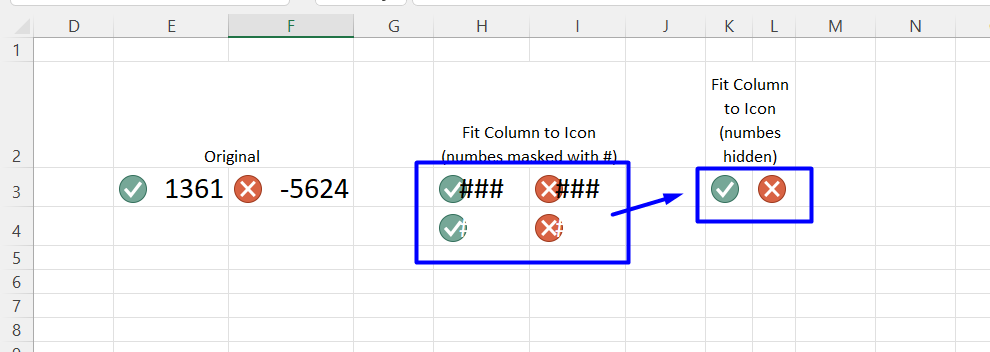## Display data in a format other than the system locale

With cloud systems and global businesses, the data is not necessarily available in a specific locale. Data, especially numbers, and dates, are stored and displayed as texts or converted in Excel.

Add the locale explicitly in the number format to display them in the specific format. With GcExcel, working with culture-specific data requires three steps, as described below:

1. set the culture info for the workbook
2. parse the numbers/dates
3. apply number format
``````workbook.Worksheets.Add().Name = "Culture";

workbook.Worksheets["Culture"].Range["A1"].Value = "Date as Text";
workbook.Worksheets["Culture"].Range["A2"].Value = "12/27/2022";
workbook.Worksheets["Culture"].Range["A3"].Value = "08/07/2022";

workbook.Culture = CultureInfo.GetCultureInfo("en-US"); //set culture of data available
workbook.AutoParse = true;

workbook.Worksheets["Culture"].Range["B1"].Value = "Date converted to system locale (not US)";
workbook.Worksheets["Culture"].Range["B2"].Value = "12/27/2022";
workbook.Worksheets["Culture"].Range["B3"].Value = "08/07/2022";

workbook.Worksheets["Culture"].Range["C1"].Value = "Formatted to US date format";
workbook.Worksheets["Culture"].Range["C2"].Value = "12/27/2022";
workbook.Worksheets["Culture"].Range["C3"].Value = "08/07/2022";
workbook.Worksheets["Culture"].Range["C2:C3"].NumberFormat = "[\$-en-US]mm-dd-yyyy";

workbook.Worksheets["Culture"].Columns.AutoFit();``````Check out the GcExcel .NET demo to apply a number format to culture-specific data!

## Turn Decimal Numbers into Fractions

Fractions are a special way to present decimal numbers. To display decimals as fractions, include a forward slash (/) in the number format code, separating the integer part with a space. For example:

• # #/# or # ##/##, depending on the number of fraction remainder digits to be shown.
• ###/###, to display an improper fraction with up to 3 digits.
• ##/N, rounding fractions to a specific denominator with Nth base

For the code snippet below, the decimals are displayed as depicted in the following image:

``````workbook.Worksheets.Add().Name = "Fractions";

workbook.Worksheets["Fractions"].Range["A1"].Value = "Decimal";
workbook.Worksheets["Fractions"].Range["B1"].Value = "# ##/##";
workbook.Worksheets["Fractions"].Range["C1"].Value = "###/###";
workbook.Worksheets["Fractions"].Range["D1"].Value = "##/4";

workbook.Worksheets["Fractions"].Range["A2:D2"].Value = 0.25;
workbook.Worksheets["Fractions"].Range["A3:D3"].Value = 5.64;
workbook.Worksheets["Fractions"].Range["A4:D4"].Value = 2.5;

workbook.Worksheets["Fractions"].Range["B2:B4"].NumberFormat = "# ##/##";
workbook.Worksheets["Fractions"].Range["C2:C4"].NumberFormat = "###/###";
workbook.Worksheets["Fractions"].Range["D2:D4"].NumberFormat = "##/4";

workbook.Worksheets["Fractions"].Columns.AutoFit();``````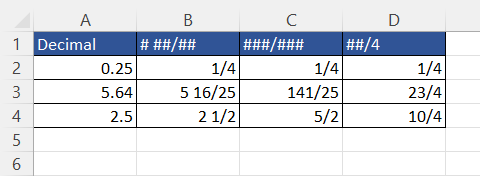## Conclusion